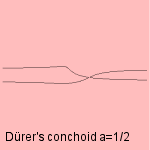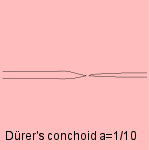# Dürer's conchoid

## quarticLet there be two points Q(q,0) and R(0,r) where the sum of q and r is constant (a). Now construct points P on line l through RQ, so that PQ is equal to a constant (b). Then the locus of P defines Dürer's conchoid. Neglecting linear transformations, the form of the curve is only dependent on the quotient b/a, which can be replaced by one parameter. The definition of the curve is a variation on the (normal) conchoid. Sometimes the curve is called Dürer's shell curve. The curve appeared in a work of Albrecht Dürer (1471 - 1528), named 'Instruction in measurement with compasses and straight edge' (1525). He only found one of the two branches of the curve. Albrecht called the curve 'ein muschellini' 1). Dürer looked at the situation that a=13, for points P and P' where PRQ and RQP' both have a length of 16 units. It can be shown that the envelope of the line P'QRP is a parabola.notes 1) Muschellini = conchoid = shell.